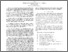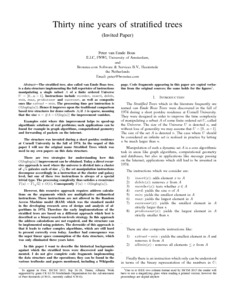# PP-2013-16: Thirty nine years of stratified trees

PP-2013-16: van Emde Boas, Peter (2013) Thirty nine years of stratified trees. [Report]Preview Text (Full Text) PP-2013-16.text.pdf Download (6MB) | PreviewText (Abstract) PP-2013-16.abstract.txt Download (2kB)

## Abstract

The stratified tree, also called van Emde Boas tree, is a data
structure implementing the full repertoire of instructions
manipulating a single subset A of a finite ordered Universe U = [0
... u-1]. Instructions include `member', `insert', `delete', `min',
`max', `predecessor' and `successor', as well as composite ones like
`extract-min'. The processing time per instruction is
O(loglog(u)). Hence it improves upon the traditional comparison based
tree structures for dense subsets A; if A is sparse, meaning that the
size n = # A = O(log(u)) the improvement vanishes.

Examples exist where this improvement helps to speed-up algorithmic
solutions of real problems; such applications can be found for example
in graph algorithms, computational geometry and forwarding of packets
on the internet.

The structure was invented during a short postdoc residence at Cornell
University in the fall of 1974. In the sequel of this paper I will use
the original name Stratified Trees which was used in my own papers on
this data structure.

There are two strategies for understanding how this O(loglog(u))
improvement can be obtained. Today a direct recursive approach is used
where the universe is divided into a cluster of sqrt(u) galaxies each
of size sqrt(u); the set manipulation instructions decompose
accordingly in a instruction at the cluster and galaxy level, but one
of these two instructions is always of a special trivial type. The
processing complexity thus satisfies a recurrence T(u) = T(sqrt(u)) +
O(1). Consequently T(u) = O(loglog(u)).

However, this recursive approach requires address calculations on the
arguments which use multiplicative arithmetical instructions. These
instructions are not allowed in the Random Access Machine model (RAM)
which was the standard model in the developing research area of design
and analysis of algorithms in 1974. Therefore the early
implementations of the stratified trees are based on a different
approach which best is described as a binary-search-on-levels
strategy. In this approach the address calculations are not required,
and the structure can be implemented using pointers. The downside of
this approach is that it leads to rather complex algorithms, which are
still hard to present correctly even today. Another bad consequence
was the super linear space consumption of the data structure, which
was only eliminated three years later.

In this paper I want to describe the historical backgrounds against
which the stratified trees were discovered and implemented. I do not
give complete code fragments implementing the data structure and the
operations; they can be found in the various textbooks and papersView Item